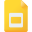# applications of derivatives tutorialsIndeterminate Forms and L’Hospital’s Rule
Applications of Derivatives, High School, United States, Differentiation, CalculusConcavity and the Second Derivative Test
Applications of Derivatives, High School, United States, Calculus, DifferentiationCurve Sketching using Calculus
Applications of Derivatives, High School, United States, Mathematics, Calculus, Curve SketchingIncreasing and Decreasing Functions and the First Derivative Test
Derivative, High School, United States, Calculus, Applications of DerivativesOptimization Problems using Derivatives
Applications of Derivatives, High School, United States, Differentiation, CalculusNewton's Method to solve high degree equations
Applications of Derivatives, High School, United States, Calculus, Newton's MethodHow Derivatives Affect the Shape of a Graph
Derivative, High School, United States, Differentiation, Applications of Derivatives, Calculus, Mathematics